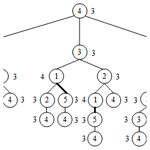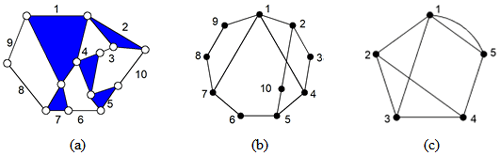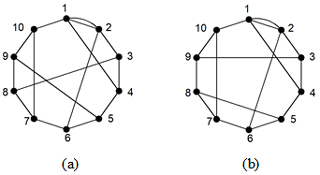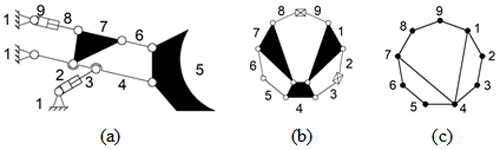Mech. Sci., 13, 371–386, 2022
https://doi.org/10.5194/ms-13-371-2022
Mech. Sci., 13, 371–386, 2022
https://doi.org/10.5194/ms-13-371-2022
Research article | 25 Apr 2022# Novel loop tree for the similarity recognition of kinematic chains

Novel loop tree for the similarity recognition of kinematic chains
Lei Wang1,2, Liang Sun1,2, Rongjiang Cui3, Yadan Xu3, Gaohong Yu1,2, and Chuanyu Wu1,2 Lei Wang et al.
• 1Faculty of Mechanical Engineering and Automation, Zhejiang Sci-Tech University, Hangzhou 310018, China
• 2Zhejiang Province Key Laboratory of Transplanting Equipment and Technology, Hangzhou 310018, China
• 3Hangzhou Vocational and Technical College, Hangzhou 310018, China

Abstract

The similarity recognition of kinematic chains (KCs) is helpful for improving the efficiency of configuration synthesis, which has been paid more and more attention in recent years. The existing recognition methods are divided into the definition method and feature constant method. Among them, the definition method is difficult to adopt in practice because of its long operation time, especially when the number of similar vertices in KCs is large. In this paper, the new concepts of a loop tree (LT) and a loop tree matrix (LTM) have been proposed, which improve the efficiency of similarity recognition. This method is applied on the complete structure of the following: 8-link with 1 DOF (degree of freedom), 9-link with 2 DOF, 10-link with 1 DOF, 12-link with 1 DOF, 13-link with 2 DOF, 14-link with 3 DOF, 15-link with 4 DOF planar single-joint KCs, and contracted graphs with up to six independent loops. All results are verified by the definition method to prove the good applicability, reliability, and efficiency of the proposed method. Simultaneously, the application case of the similarity recognition in a mechanism creation is given to provide a reference for an innovative design.

Share
1 Introduction

The similarity recognition of kinematic chains (KCs) is an important part of the mechanism of innovation design, which can avoid isomorphism in the synthesis process and reduce the generation of redundant design schemes (Yan, 1992; Tsai, 2000; Sun et al., 2022). At present, relatively little research has been done on similarity recognition, including the definition method and the characteristic constant method. Among them, similarity recognition is accurate when using the definition method of graph theory, but numerous computations will be generated, especially when the number of links exceeds 10 (Sun et al., 2020). And for the characteristic constant method, most of them are not dedicated to similarity recognition but are more often applied to isomorphism recognition. Similarity recognition and isomorphism detection are essentially the same. The difference lies in that one is a recognition within graphs and the other is a recognition between graphs. Therefore, the characteristic constant method applied to isomorphism detection can also be used for similarity recognition.

Vertices in graph theory represent KC components, and a similarity analysis of the KC components can be transformed into a similarity recognition problem of vertices in topological graphs. Harary and Palmar (1966) discussed graph similarity, where it is noted that, if vertices u and v of graph G are similar, then $G-u\cong G-v$ and vice versa. Freudenstein (1967) provided the condition for similarity from the perspective of the permutation cycle of the automorphism group. However, the calculation amount is substantially larger, and a KC with n links requires n! cycle times. Lv (1989) verified the necessary conditions for similar vertices. Wang and Yan (2002) classified the similarity between components into symmetrical, parallel, transferred, and irregular states. This method only uses the vertex degree of the KC and does not reflect the specific connection between the components and misjudgments in several special cases. Sun et al. (2020) proposed similarity recognition methods based on the definition of similarity. However, the judgment is complicated when the number of similar vertices in the KCs are large.

The rest of this paper is organized as follows. Section 2 proposes the concept of the LT and LTM. Section 3 applies the LT and LTM to similarity recognition and gives some examples. Section 4 introduces the similarity algorithm application. Section 5 analyzes the recognition results. Finally, Sect. 6 concludes the paper.

2 Concepts of LT and LTM

## 2.1 LT

Set A is a vertex of graph G. All loops with A as the starting vertex are searched and given in the form of a tree (where vertex A is the root of the tree). For example, in Fig. 1c, the LT with vertex 4 is as shown in Fig. 2 (thick, solid lines in LT represent parallel edges 1–5).Figure 1(a) A 10-link with 1 DOF (degree of freedom) KC, (b) a topological graph, and (c) a contracted graph.

The LT represents all the information of vertices in the graph, such as the vertex degree information, assignment information of connecting edges, and loop information. If the LTs of two vertices are the same, then the statuses of the two vertices in the graph are equivalent. For example, in Fig. 1c, the LT has vertex 1 as the root, as shown in Fig. 3. Although the tree structure is the same as that in Fig. 2, the vertex degree and the assignment information of connection edges are inconsistent; thus, vertices 4 and 1 are non-similar.

## 2.2 LTM

For the convenience of computer implementation, a matrix expression of LT is proposed, as shown in Table 1. Each number in a loop contains three messages. The first message is the degree of the root, the second message specifies the number of branch edges, and the third message is the value assigned for branch edges (fine and thick solid lines are assigned 1 and 2, respectively). But when a cycle is completed, there is a last number containing only one piece of information (i.e., the degree of the root). For example, the loop 4-2-1-3-4 in Fig. 2 can be expressed as 331, 321, 421, 311, and 3. Other loops can then be expressed, and the sequence of the numbers is converted into a matrix form. The number of digits is insufficient to fill 0. The LTM of Fig. 2 is shown in Eq. (1).

Definition 1. The row elements of the LTM are sorted in descending order (i.e., Ld). For example, Ld of Fig. 2 is presented as follows:

$\begin{array}{}\text{(1)}& \begin{array}{rl}& \mathrm{LTM}=\left[\begin{array}{rrrrrr}\mathrm{331}& \mathrm{321}& \mathrm{421}& \mathrm{311}& \mathrm{3}& \mathrm{0}\\ \mathrm{331}& \mathrm{321}& \mathrm{422}& \mathrm{311}& \mathrm{3}& \mathrm{0}\\ \mathrm{331}& \mathrm{321}& \mathrm{321}& \mathrm{412}& \mathrm{311}& \mathrm{3}\\ \mathrm{331}& \mathrm{321}& \mathrm{321}& \mathrm{3}& \mathrm{0}& \mathrm{0}\\ \mathrm{331}& \mathrm{321}& \mathrm{421}& \mathrm{311}& \mathrm{3}& \mathrm{0}\\ \mathrm{331}& \mathrm{321}& \mathrm{422}& \mathrm{311}& \mathrm{3}& \mathrm{0}\\ \mathrm{331}& \mathrm{321}& \mathrm{321}& \mathrm{412}& \mathrm{311}& \mathrm{3}\\ \mathrm{331}& \mathrm{321}& \mathrm{321}& \mathrm{3}& \mathrm{0}& \mathrm{0}\\ \mathrm{331}& \mathrm{312}& \mathrm{421}& \mathrm{321}& \mathrm{311}& \mathrm{3}\\ \mathrm{331}& \mathrm{312}& \mathrm{421}& \mathrm{321}& \mathrm{3}& \mathrm{0}\\ \mathrm{331}& \mathrm{312}& \mathrm{421}& \mathrm{321}& \mathrm{311}& \mathrm{3}\\ \mathrm{331}& \mathrm{312}& \mathrm{421}& \mathrm{321}& \mathrm{3}& \mathrm{0}\end{array}\right],\\ & {L}_{\mathrm{d}}=\left[\begin{array}{rrrrrr}\mathrm{331}& \mathrm{321}& \mathrm{422}& \mathrm{311}& \mathrm{3}& \mathrm{0}\\ \mathrm{331}& \mathrm{321}& \mathrm{422}& \mathrm{311}& \mathrm{3}& \mathrm{0}\\ \mathrm{331}& \mathrm{321}& \mathrm{421}& \mathrm{311}& \mathrm{3}& \mathrm{0}\\ \mathrm{331}& \mathrm{321}& \mathrm{421}& \mathrm{311}& \mathrm{3}& \mathrm{0}\\ \mathrm{331}& \mathrm{321}& \mathrm{321}& \mathrm{412}& \mathrm{311}& \mathrm{3}\\ \mathrm{331}& \mathrm{321}& \mathrm{321}& \mathrm{412}& \mathrm{311}& \mathrm{3}\\ \mathrm{331}& \mathrm{321}& \mathrm{321}& \mathrm{3}& \mathrm{0}& \mathrm{0}\\ \mathrm{331}& \mathrm{321}& \mathrm{321}& \mathrm{3}& \mathrm{0}& \mathrm{0}\\ \mathrm{331}& \mathrm{312}& \mathrm{421}& \mathrm{321}& \mathrm{311}& \mathrm{3}\\ \mathrm{331}& \mathrm{312}& \mathrm{421}& \mathrm{321}& \mathrm{311}& \mathrm{3}\\ \mathrm{331}& \mathrm{312}& \mathrm{421}& \mathrm{321}& \mathrm{3}& \mathrm{0}\\ \mathrm{331}& \mathrm{312}& \mathrm{421}& \mathrm{321}& \mathrm{3}& \mathrm{0}\end{array}\right].\end{array}\end{array}$
3 Similarity recognition

## 3.1 Similarity recognition algorithm

If Ld of two vertices are the same, then the two vertices are judged to be similar; otherwise, they are non-similar. The steps of the similarity recognition algorithm are as follows.

First calculate Ld of each vertex and compare the Ld parameters of two vertices. If Ld parameters of two vertices are different, then these vertices are non-similar, and the next vertices would be considered. Otherwise, these vertices are similar. The next vertices are then considered until all candidate vertices are identified. For example, vertices 2 and 3 in Fig. 1c have the same Ld as shown in Eq. (2); thus, vertices 2 and 3 are similar.

$\begin{array}{}\text{(2)}& {L}_{\mathrm{d}\mathrm{2}}={L}_{\mathrm{d}\mathrm{3}}=\left[\begin{array}{rrrrrr}\mathrm{331}& \mathrm{422}& \mathrm{311}& \mathrm{321}& \mathrm{311}& \mathrm{3}\\ \mathrm{331}& \mathrm{422}& \mathrm{311}& \mathrm{321}& \mathrm{3}& \mathrm{0}\\ \mathrm{331}& \mathrm{421}& \mathrm{321}& \mathrm{311}& \mathrm{3}& \mathrm{0}\\ \mathrm{331}& \mathrm{421}& \mathrm{321}& \mathrm{3}& \mathrm{0}& \mathrm{0}\\ \mathrm{331}& \mathrm{321}& \mathrm{422}& \mathrm{311}& \mathrm{311}& \mathrm{3}\\ \mathrm{331}& \mathrm{321}& \mathrm{421}& \mathrm{3}& \mathrm{0}& \mathrm{0}\\ \mathrm{331}& \mathrm{321}& \mathrm{321}& \mathrm{411}& \mathrm{3}& \mathrm{0}\\ \mathrm{331}& \mathrm{321}& \mathrm{321}& \mathrm{312}& \mathrm{411}& \mathrm{3}\\ \mathrm{331}& \mathrm{321}& \mathrm{321}& \mathrm{3}& \mathrm{0}& \mathrm{0}\\ \mathrm{331}& \mathrm{321}& \mathrm{321}& \mathrm{3}& \mathrm{0}& \mathrm{0}\\ \mathrm{331}& \mathrm{321}& \mathrm{312}& \mathrm{421}& \mathrm{311}& \mathrm{3}\\ \mathrm{331}& \mathrm{321}& \mathrm{312}& \mathrm{421}& \mathrm{3}& \mathrm{0}\end{array}\right].\end{array}$

## 3.2 Illustrative examples of similarity recognition

The proposed method is tested on planar single-joint KCs (PSKCs) and contracted graphs of KCs to prove its versatility. Illustrative examples are provided as follows.

### 3.2.1 Examples of PSKCs

Example 1. In total, two 10-link PSKCs are considered in Fig. 4a and b.

The adjacency matrices of two 10-link PSKCs in Fig. 4 are entered into the similarity recognition programs. The Ld of each vertex is solved and compared with other vertices of same graph. Similar information to that in Fig. 4 is shown in Table 2. To some extent, solving the similarity of the graphs can also identify whether they are isomorphic. Because the similar information in Fig. 4a and b is different, it can be judged that they are not isomorphic.

Example 2. In total, two 28-link PSKCs are considered in Fig. 5a and b, as discussed in Uicker and Raicu (1975).

The adjacency matrices of two 28-link PSKCs in Fig. 5 are entered into the similarity recognition programs. Similar information to that in Fig. 5 is shown in Table 3. Because the similar information of Fig. 5a and b is the same, there is the possibility of isomorphism between two graphs.

### 3.2.2 Examples of contracted graphs

The distinction of contracted graphs lies in the existence of parallel edges, which can be distinguished by setting up the parallel edge value equal to the number of parallel edges. The similarity recognition method is same as the single-hinged KC. As shown in Fig. 8, two 10-link contracted graphs are considered.

The adjacency matrices of two 10-link contracted graphs in Fig. 6 are entered into the similarity recognition programs. Similar information to that in Fig. 6 is shown in Table 4. Because the similar information of Fig. 6a and b is different, it can be judged that they are not isomorphic.Figure 6(a) A 10-link contracted graph (1) and (b) a 10-link contracted graph (2).

4 Similarity algorithm application

## 4.1 KC similarity recognition application

In addition to the examples in Sect. 3, the similarity recognition algorithm is applied to the complete atlas of 10-link with 1 DOF PSKCs. The recognition results are consistent with the definition method of the graph theory, as shown in Appendix A (only the upper triangular elements of the adjacency matrix are given in the Appendix A).

## 4.2 Innovative mechanism design

Yan (1992) proposed a regenerated KC method for innovative mechanism design, which represents logical reasoning for the KC regeneration process. In logical reasoning, the similarity of the KC components should be analyzed first to reduce redundant design schemes and improve the innovative design efficiency. Vertices in the graph theory represent KC components, and a similar analysis of the KC components can be transformed into a similarity recognition problem of vertices in topological graphs.

The working device of a loader is the main part for realizing the shoveling operation and material loading. Its structure directly affects the working size and comprehensive performance of the loader. Taking the mechanism's innovative design of a 6-link loader as an example, as shown in Fig. 7, the application of similarity recognition in mechanism innovative design is illustrated.Figure 7(a) Loader functional schematic, (b) structural representation, and (c) topological graph representation with the (1) rack, (2, 3) rotary bucket oil cylinder, (4) moving arm, (5) bucket, (6) connecting rod, (7) rocker, and (8, 9) lifting oil cylinder.

Step 1. Retrieve the existing design by task and enumerate all the KCs.

Figure 7c shows the original mechanism of the loader's innovative design. According to Tsai (2000), 35 9-link with 2 DOF topological graphs are obtained.

Step 2. Analyze the structural and functional constraints of the original mechanism and conducting topological graphs screening. For example, see the structural and functional constraints of Fig. 7a.

1. There must be a ternary link as a rack.

2. There must be two oil cylinders as the original moving parts, with one as the rotating oil cylinder and one as the lifting oil cylinder, where one end of the lifting oil cylinder is connected to the rack, and the other one is connected to the moving arm. It shows that the topology graph has at least two binary chains of length 2.

3. There must be a binary link as the output bucket, where one end is connected with the moving arm but cannot be directly connected with the rack.

4. There must be a moving arm, for which the one end is connected to the rack, and the other end is connected to the output bucket. One end of the lifting cylinder and the rocker should be connected with the moving arm by considering the coordination and stiffness of the mechanism.

The direct connection between the binary link and hydraulic cylinder should be avoided to overcome the excessive forces on the local components of the transmission. These excessive forces can create the imbalance. The topology graphs that fulfill the above constraint criteria are shown in the first column of Table 5.

Step 3. Specify the topology graphs that meet the structural and functional requirements of the mechanism. For example, see the specialization of Fig. 7c.

First, determine the rack. Both vertex 1 and vertex 7 are three degree vertices and can be used as racks. Vertices 1 and 7 are similar, according to the loop tree matrix, and choosing vertex 1 or vertex 7 as racks is equivalent. In this paper, vertex 1 is chosen as a rack.

Second, the original moving parts are determined. The original moving parts are binary chains of length 2 in topology graph. The edges are assigned to the two that attached to the rack. Binary chain 23 and 56 are no longer similar, according to the loop tree matrix. The binary chains 89 and 23, 89 and 56, and 56 and 23 can be chosen as the original moving parts.

When binary chains 89 and 23 are chosen as the original moving parts, only vertex 5 can be chosen as the output bucket. The final functional schematic is shown in Table 5.

When binary chains 56 and 23 are chosen as the original moving parts, only vertex 5 can be chosen as the output bucket. The final functional schematic is shown in Fig. 7a.

When binary chains 56 and 89 are chosen as the original moving parts, it does not satisfy the structural constraint (4).

According to the above specialization process, other topology graphs are given specifically, as shown in the second column of Table 5. In specialized topological graph, represents the rack, represents output the bucket, represents the moving arm, and the solid lines represent prismatic pair.

Step 4. Draw the schematic graph and delete the existing design as the rest forms the new designs, as shown in the third column of Table 5.

5 Recognition result analysis

At present, there are relatively few studies on the similarity, and the recognition methods are difficult when attempting to meet the requirements in reliability and efficiency. In this paper, a similarity recognition method based on the tree loop was proposed, which aims to provide some theoretical support for configuration synthesis and innovation.

## 5.1 Computational complexity

Compared with the graph theory definition method for recognizing similar vertices, the application of the LT method can reduce the computer computation considerably. Figure 8 shows the available methods for identifying similar vertices, including the definition method needs a calculation of 28! cycle times. The method of Dharanipragada and Chintada (2016) requires a calculation of $\mathrm{16}\mathrm{!}×\mathrm{8}\mathrm{!}×\mathrm{4}\mathrm{!}$ cycle times, and the LT method only needs $\sum _{\mathrm{1}}^{\mathrm{16}}\mathrm{1}+\sum _{\mathrm{1}}^{\mathrm{8}}\mathrm{1}+\sum _{\mathrm{1}}^{\mathrm{4}}\mathrm{1}$ cycle times.

## 5.2 Computational complexity

LTM contains almost all information of the LT, such as the vertex degree information, assignment information of connecting edges, and loop information. Therefore, the LT method used to recognize the similar vertices is effective, as proven by numerous recognition examples.

6 Conclusions

In this paper, the concept of a loop tree containing multidimensional topological information is proposed, and the loop tree matrix is established for similarity recognition. This method is applied to planar single-joint KCs and contracted graphs to reflect its applicability, and its accuracy is verified by the definition method. At the same time, the efficiency of the method is proved by qualitatively comparing the calculation cycle times of various similarity recognition methods. Finally, through the application of the similarity recognition in mechanism creation, the practical significance of this method is shown, which provides a reference for mechanical equipment design.

Code and data availability

Code and data can be made available upon request.

Author contributions

LW was responsible for the conceptualization, methodology, and preparing the original draft. RC and YX curated the data, reviewed and edited the paper, developing the software, and validating the research. LS, GY, and CW took responsibility for supervising and the project administration. All authors have read and agreed to the published version of the paper.

Competing interests

The contact author has declared that neither they nor their co-authors have any competing interests.

Disclaimer

Publisher’s note: Copernicus Publications remains neutral with regard to jurisdictional claims in published maps and institutional affiliations.

Financial support

This work has been supported in part by the National Natural Science Foundation of China (grant no. 51975534), the Zhejiang Provincial Key Research and Development Program (grant no. 2022C02006), the high-level talents research start-up project of the Hangzhou Vocational and Technical College (grant no. HZYGCC202103), and the Public Welfare Technology Application Research Project of Zhejiang Province (grant no. LGN21E050001).

Review statement

This paper was edited by Daniel Condurache and reviewed by two anonymous referees.

References

Ambekar, A. G. and Agrawal, V. P.: Canonical numbering of kinematic chains and isomorphism problem: min code, Mech. Mach. Theory, 22, 453–461, 1987.

Cubillo, J. P. and Wan, J.: Comments on mechanism kinematic chain isomorphism identification using adjacent matrices, Mech. Mach. Theory, 40, 131–139, 2005.

Dharanipragada, V., and Chintada, M.: Split Hamming String as an Isomorphism Test for One Degree-of-Freedom Planar Simple-Jointed Kinematic Chains Containing Sliders, J. Mech. Design, 138, 082301, https://doi.org/10.1115/1.4033611, 2016.

Freudenstein, F.: The basic concepts of Polya's theory of enumeration, with application to the structural classification of mechanisms, J. Mechanisms, 2, 275–290, 1967.

Harary, F. and Palmer, E. M.: On similar points of a graph, J. Math. Mech., 15, 623–630, 1966.

He, P. R., Zhang, W. J., and Li, Q.: Some further development on the eigensystem approach for graph isomorphism detection, J. Frankl. Inst., 342, 657–673, 2005.

Lv, T.: Sufficient and Necessary Conditions for Similarity, J. Beijing Univ. Technol., 15, 63–66, 1989.

Mruthyunjaya, T. S.: A computerized methodology for structural synthesis of kinematic chains: Part 1 – Formulation, Mech. Mach. Theory, 19, 487–495, 1984.

Mruthyunjaya, T. S. and Balasubramanian, H. R.: In quest of a reliable and efficient computational test for detection of isomorphism in kinematic chains, Mech. Mach. Theory, 22, 131–139, 1987.

Rai, R. K. and Punjabi, S.: Kinematic chains isomorphism identification using link connectivity number and entropy neglecting tolerance and clearance, Mech. Mach. Theory, 123, 40–65, 2018.

Rai, R. K. and Punjabi, S.: A new algorithm of links labelling for the isomorphism detection of various kinematic chains using binary code, Mech. Mach. Theory, 131, 1–32, 2019.

Rao, A. C. and Rao, C. N.: Loop based pseudo hamming values – I testing isomorphism and rating kinematic chains, Mech. Mach. Theory, 28, 113–127, 1993.

Rao, A. C. and Varada Raju, D.: Application of the hamming number technique to detect isomorphism among kinematic chains and inversions, Mech. Mach. Theory, 26, 55–75, 1991.

Rao, Y. V. D. and Rao, A. C.: Generation of Epicyclic Gear Trains of One Degree of Freedom, J. Mech. Design, 130, 232–245, 2008.

Shin, J. K. and Krishnamurty, S.: Development of a standard code for colored graphs and its application to kinematic chains, International Design Engineering Technical Conferences and Computers and Information in Engineering Conference, American Society of Mechanical Engineers, 9419, 247–254, 1992.

Sun, L., Cui, R., Ye, Z., Zhou, Y., and Wu, C.: Similarity recognition and isomorphism identification of planar kinematic chains, Mech. Mach. Theory, 145, 103678, https://doi.org/10.1016/j.mechmachtheory.2019.103678, 2020.

Sun, L., Ye, Z., Cui, R., Huang, X., and Wu, C.: Eliminating isomorphism identification method for synthesizing nonfractionated kinematic chains based on graph similarity, Mech. Mach. Theory, 167, 104500, https://doi.org/10.1016/j.mechmachtheory.2021.104500, 2022.

Sun, W., Kong, J., and Sun, L.: The improved hamming number method to detect isomorphism for kinematic chain with multiple joints, J. Adv. Mech. Des. Syst., 11, JAMDSM0061, https://doi.org/10.1299/jamdsm.2017jamdsm0061, 2017.

Sunkari, R. P. and Schmidt, L. C.: Reliability and Efficiency of the Existing Spectral Methods for Isomorphism Detection, J. Mech. Design, 128, 1246–1252, 2006.

Tang, C. and Liu, T.: The Degree Code – A New Mechanism Identifier, J. Mech. Design, 115, 627–630, 1993.

Tsai, L. W.: Mechanism Design: Enumeration of Kinematic Structures According to Function, Appl. Mech. Rev., 122, B85–B86, 2000.

Uicker Jr., J. J. and Raicu, A.: A method for the identification and recognition of equivalence of kinematic chains, Mech. Mach. Theory, 10, 375–383, 1975.

Venkata Kamesh, V., Mallikarjuna Rao, K., and Annambhotla, B. S. R.: An Innovative Approach to Detect Isomorphism in Planar and Geared Kinematic Chains Using Graph Theory, J. Mech. Design, 139, 122301, https://doi.org/10.1115/1.4037628, 2017.

Wang, Y. X. and Yan, H. S.: Computerized rules-based regeneration method for conceptual design of mechanisms, Mech. Mach. Theory, 37, 833–849, 2002.

Yadav, J. N., Pratap, C. R., and Agrawal, V. P.: Computer aided detection of isomorphism among binary chains using the link-link multiplicity distance concept, Mech. Mach. Theory, 31, 873–877, https://doi.org/10.1016/0094-114X(96)00002-X, 1996.

Yan, H. S.: A methodology for creative mechanism design, Mech. Mach. Theory, 27, 235–242, 1992.

Yan, H. S. and Hall, A. S.: Linkage Characteristic Polynomials: Assembly Theorems, Uniqueness, J. Mech. Design, 104, 11–20, 1982.